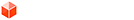## [code] Calculate volume on manifold surface without grouping

### [code] Calculate volume on manifold surface without grouping

The question has been often raised on how to get the volume of manifold surface without temporarily grouping and then exploding it.

With the release of SU2017 things got more difficult because the old references before the group and explode are no longer valid. (This happens because of persistant_id implementations in the SketchUp Core.)

@TIG pointed me toward an old thread on SketchUcation (hiding in the "Plugins" forum) in which …
@AdamB proposed a totally mathematical solution, without a temporary group.

Following is my rendition of AdamB's "no temporary group" solution.

Mine does not modify API class Geom::Point3d, but converts to an Array which already has a #dot method.

Mine also uses #grep which is very fast when comparing class identity, ... instead of calling #is_a? on each and every object in the geometry collection on the Ruby-side, during each iteration.

Code: Select all
`# calculate_volume(geometry)# Calculate volume on a set of faces without a temporary group.## Based on an old SCF topic thread post by AdamB:# http://sketchucation.com/forums/viewtopic.php?p=14598#p14598## @param geometry [Array<Drawingelement>] Array of collected geometry references.# @return [Float] the computed volume if successful, 0.0 if not.def calculate_volume(geometry)  geometry.grep(Sketchup::Face).map {|f|    (2 * f.area * (f.vertices.position.to_a.dot(f.normal))) / 6        }.reduce(:+)rescue => e  puts e.inspect  return 0.0end`

If you are still using Ruby 1.8, you'll need to replace .reduce(:+) with .inject {|sum,n| sum+n } in the code above, like so ...

Code: Select all
`# calculate_volume(geometry)# Calculate volume on a set of faces without a temporary group.## Based on an old SCF topic thread post by AdamB:# http://sketchucation.com/forums/viewtopic.php?p=14598#p14598## @param geometry [Array<Drawingelement>] Array of collected geometry references.# @return [Float] the computed volume if successful, 0.0 if not.def calculate_volume(geometry)  geometry.grep(Sketchup::Face).map {|f|    (2 * f.area * (f.vertices.position.to_a.dot(f.normal))) / 6        }.inject {|sum,n| sum+n }rescue => e  puts e.inspect  return 0.0end`

Bonus method for a volume display string in model units:
See: [code] format volume in model units
1
I'm not here much anymore. But a PM will fire email notifications.Dan Rathbun
PluginStore Author